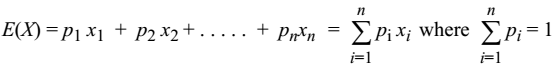If X denotes a discrete random variable which can assume the values x1, x2, ..., xn with respective probabilities p1, p2, ..., pn then the mathematical expectation of X, denoted by E(X) is defined byThe mathematical Expectation E(X) of a random variable is simply the arithmetic mean.

Result

If ϕ(X) is a function of the random variable X,

then E[ϕ(X)] = ∑ P(X = x) ϕ(x)